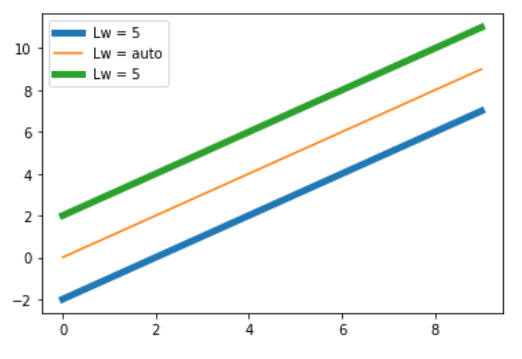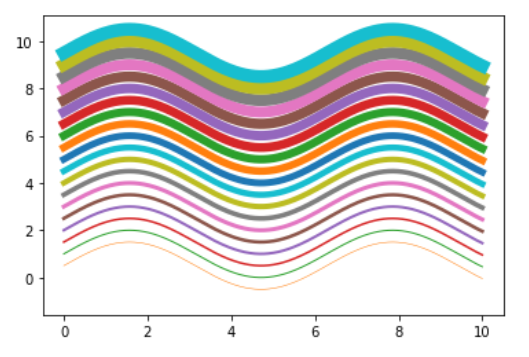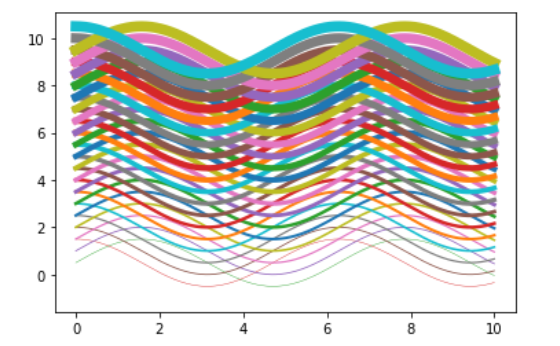# How to Change the Line Width of a Graph Plot in Matplotlib with Python?

• Last Updated : 12 Nov, 2020

Prerequisite : Matplotlib

In this article we will learn how to Change the Line Width of a Graph Plot in Matplotlib with Python. For that one must be familiar with the given concepts:

• Matplotlib : Matplotlib is a tremendous visualization library in Python for 2D plots of arrays. Matplotlib may be a multi-platform data visualization library built on NumPy arrays and designed to figure with the broader SciPy stack. It was introduced by John Hunter within the year 2002.
• Graph Plot : A plot is a graphical technique for representing a data set, usually as a graph showing the relationship between two or more variables.
• Line Width : The width of a line is known as line width. One can change the line width of a graph in matplotlib using a feature.

### Approach

• Import packages
• Import or create the data
• Draw a graph plot with a line
• Set the line width by using line-width feature ( lw can also be used as short form ).

Example 1:

## Python3

 `# importing packages``import` `matplotlib.pyplot as plt``import` `numpy as np`` ` `# create data``x_values ``=` `np.arange(``0``, ``10``)``y_values ``=` `np.arange(``0``, ``10``)`` ` `# Adjust the line widths``plt.plot(x_values, y_values ``-` `2``, linewidth``=``5``)``plt.plot(x_values, y_values)``plt.plot(x_values, y_values ``+` `2``, lw``=``5``)`` ` `# add legends and show``plt.legend([``'Lw = 5'``, ``'Lw = auto'``, ``'Lw = 5'``])``plt.show()`

Output :Example 2 :

## Python3

 `# importing packages``import` `matplotlib.pyplot as plt``import` `numpy as np`` ` `# create data``x_values ``=` `np.linspace(``0``, ``10``, ``1000``)``y_values ``=` `np.sin(x_values)`` ` `# Adjust the line widths``for` `i ``in` `range``(``20``):``    ``plt.plot(x_values, y_values ``+` `i``*``0.5``, lw``=``i``*``0.5``)``     ` `plt.show()`

Output :Example 3 :

## Python3

 `# importing packages``import` `matplotlib.pyplot as plt``import` `numpy as np`` ` `# create data``x_values ``=` `np.linspace(``0``, ``10``, ``1000``)`` ` `# Adjust the line widths``for` `i ``in` `range``(``20``):``    ``plt.plot(x_values, np.sin(x_values) ``+` `i``*``0.5``, lw``=``i``*``0.4``)``    ``plt.plot(x_values, np.cos(x_values) ``+` `i``*``0.5``, lw``=``i``*``0.4``)``     ` `plt.show()`

Output :My Personal Notes arrow_drop_up